﻿ Beam Formulas

# Beam Formulas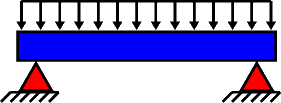Beam formulas may be used to determine the deflection, shear and bending moment in a beam based on the applied loading and boundary conditions.

## Reference Documents:

1. Formulas for Stress and Strain, Roark & Young, McGraw-Hill
2. Formulas for Stress, Strain, and Structural Matrices, Pilkey, John Wiley & Sons.

## Common Beam Formulas:PINNED-PINNED BEAM WITH UNIFORM LOAD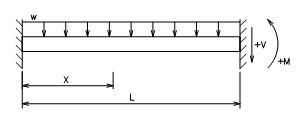FIXED-FIXED BEAM WITH UNIFORM LOAD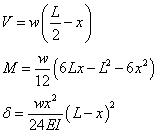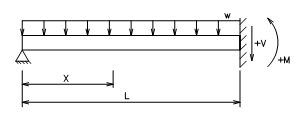PINNED-FIXED BEAM WITH UNIFORM LOAD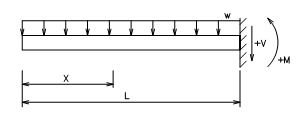FREE-FIXED BEAM WITH UNIFORM LOAD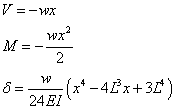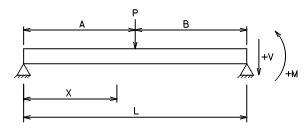PINNED-PINNED BEAM WITH POINT LOAD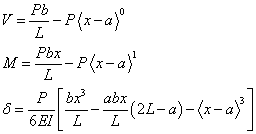See definitions of < > step functions below.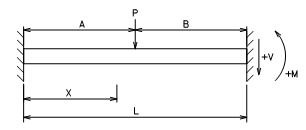FIXED-FIXED BEAM WITH POINT LOAD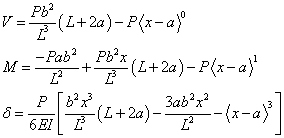See definitions of < > step functions below.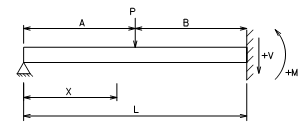PINNED-FIXED BEAM WITH POINT LOAD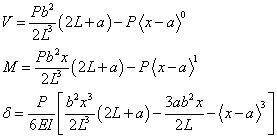See definitions of < > step functions below.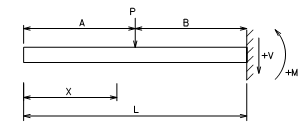FREE-FIXED BEAM WITH POINT LOAD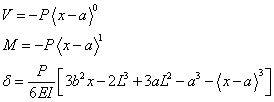See definitions of < > step functions below. Legend: w = uniform load (force/length units) V = shear M = moment d = deflection E = modulus of elasticity I = moment of inertia Definitions of step functions: If x < a then < x - a >0 = 0 If x < a then < x - a >n = 0 If x > a then < x - a >0 = 1 If x > a then < x - a >n = ( x - a )n